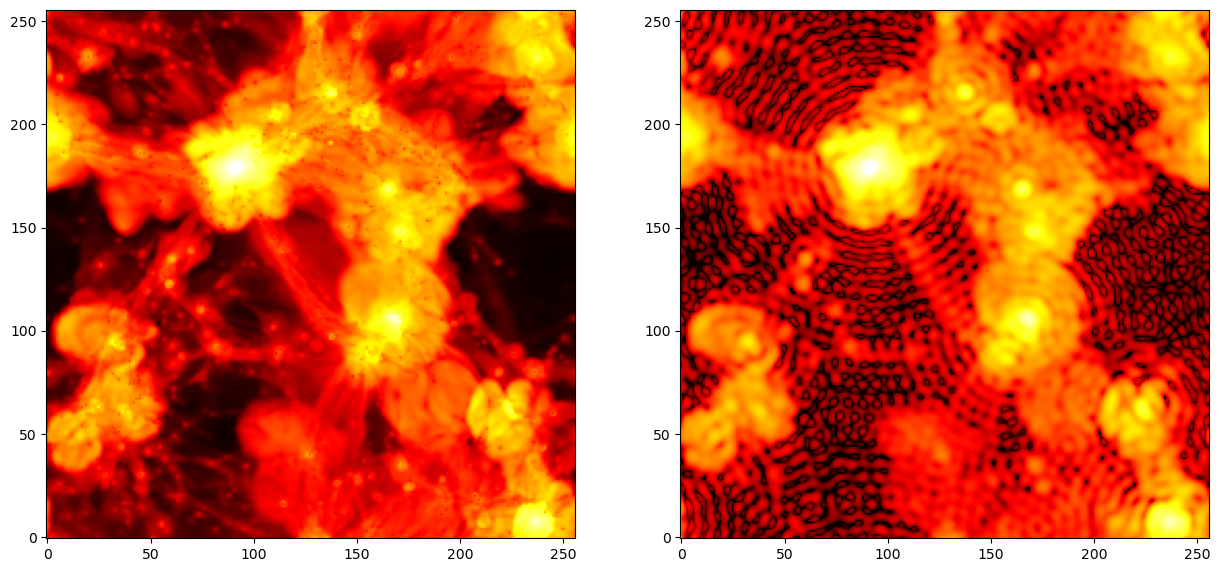# Smoothing

Pylians provides routines to smooth fields with several filters. The ingredients needed are:

• `field`. This is a 3D float numpy array that contains the input field to be smoothed.

• `BoxSize`. This is the size of the box with the input density field.

• `R`. This is the smoothing scale. This argument only matter for filters operating in configuration space: `Top-Hat` and `Gaussian`. For other filters, set this value to `0`.

• `grid`. This is the grid size of the input field, i.e. `field.shape`.

• `threads`. Number of openmp threads to be used.

• `Filter`. Filter to use. `'Top-Hat'`, `'Gaussian'` or `'Top-Hat-k'`.

• `kmin`. The minimum value of the wavenumber when using the `Top-Hat-k` filter. This argument only need to be specified when using this filter.

• `kmax`. The maximum value of the wavenumber when using the `Top-Hat-k` filter. This argument only need to be specified when using this filter.

• `W_k`. This is a 3D complex64 numpy array containing the Fourier-transform of the filter. Notice that when smoothing a discrete field, like the one stored on a regular grid, the Fourier-transform of the filter need to be computed in the same way as the for the field, i.e. through DFT instead of FT.

An example is this

```import smoothing_library as SL

BoxSize = 75.0 #Mpc/h
R       = 5.0  #Mpc.h
grid    = field.shape
Filter  = 'Top-Hat'

# compute FFT of the filter
W_k = SL.FT_filter(BoxSize, R, grid, Filter, threads)

# smooth the field
```

Pylians can also smooth 2D maps by adding `_2D` to the smoothing routines:

```import smoothing_library as SL
import numpy as np

# we take as field a temperature image from the CAMELS Multifield Dataset
f_map = 'CMD/2D_maps/data/Maps_T_IllustrisTNG_CV_z=0.00.npy'
field = np.load(f_map) #only take the first image

BoxSize = 25.0 #Mpc/h
grid    = field.shape
R       = 0.0 #only matter for configuration space filters
Filter  = 'Top-Hat-k'